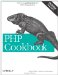# Recipe 19.6. Localizing Currency Values

#### 19.6.1. Problem

You want to display currency amounts in a locale-specific format.

#### 19.6.2. Solution

Use the money_format( ) function to produce an appropriately formatted string. Example 19-15 shows a few of the format characters that money_format( ) understands.

##### Formatting with money_format( )

 ``

Example 19-15 prints:

`5549.3 @ %i = USD 5,549.30 -25.95 @ %i = -USD 25.95 5549.3 @ %n = \$5,549.30 -25.95 @ %n = -\$25.95 5549.3 @ %+n = \$5,549.30 -25.95 @ %+n = -\$25.95 5549.3 @ %(n = \$5,549.30 -25.95 @ %(n = (\$25.95)`

money_format( ) is not available on Windows or before PHP 4.3.0. If you can't use money_format( ), use the pc_format_currency( ) function, shown in Example 19-17, to produce an appropriately formatted string. Example 19-16 shows pc_format_currency( ) in action.

##### Using pc_format_currency( )

 ` `

Example 19-16 prints:

`-12.345.678,45 TL`

#### 19.6.3. Discussion

money_format( ) is similar to sprintf( ) or strftime( )'you give it a formatting string and a value. Special sequences in the formatting string indicate how the value is formatted. Just as sprintf( ) requires the components of a format sequence to be in a particular order'percent sign, padding, type indicator, and so on'money_format( ) requires that each format sequence be in a particular order: percent sign, flags, width, left precision, right precision, conversion character. Only the percent sign and the conversion character are mandatory. Table 19-1 lists the format characters that money_format( ) understands.

##### Table 19-1. Format characters for money_format( )

Category

Format character

Description

Flag

=c

Use the single character c as a fill character. The default is space.

Flag

^

Don't use grouping characters.

Flag

+

Use locale-specified + and - characters to format positive and negative numbers. This is the default if neither the + or ( flag is used.

Flag

(

Surround negative numbers with (and ).

Flag

!

Don't use the currency symbol in the output string.

Flag

-

Left-justify all fields. If this is not present, fields are right justified.

Width

width

Make the minimum field width width. Default minimum field with is 0.

Left precision

#precision

If there are less than precision digits to the left of the decimal point, then the fill character is used to pad the width.

Right precision

.precision

The digits to the right of the decimal point are rounded to precision places before being formatted. If precision is 0, then neither a decimal point nor digits to the right of it are printed. The default is locale specific.

Conversion character

i

Use the international currency format. This usually means that a three-character code such as USD is used for the currency symbol.

Conversion character

n

Use the national currency format. This usually means that a locale-appropriate value, such as \$, is used for the currency symbol.

Conversion character

%

A literal %.

Because money_format( ) relies on the strfmon( ) system function, it is only available when that system function is available. Windows does not provide the strfmon( ) system function.

The pc_format_currency( ) function, shown in Example 19-17, gets the currency formatting information from localeconv( ) and then uses number_format( ) and some logic to construct the correct string.

##### pc_format_currency

 `= 0 ? if (1 == \$sign) { \$sign_symbol = 'positive_sign'; \$cs_precedes = 'p_cs_precedes'; \$sign_posn = 'p_sign_posn'; \$sep_by_space = 'p_sep_by_space'; } else { \$sign_symbol = 'negative_sign'; \$cs_precedes = 'n_cs_precedes'; \$sign_posn = 'n_sign_posn'; \$sep_by_space = 'n_sep_by_space'; } if (\$a[\$cs_precedes]) { if (3 == \$a[\$sign_posn]) { \$currency_symbol = \$a[\$sign_symbol].\$currency_symbol; } elseif (4 == \$a[\$sign_posn]) { \$currency_symbol .= \$a[\$sign_symbol]; } // currency symbol in front if (\$a[\$sep_by_space]) { \$amt = \$currency_symbol.' '.\$amt; } else { \$amt = \$currency_symbol.\$amt; } } else { // currency symbol after amount if (\$a[\$sep_by_space]) { \$amt .= ' '.\$currency_symbol; } else { \$amt .= \$currency_symbol; } } if (0 == \$a[\$sign_posn]) { \$amt = "(\$amt)"; } elseif (1 == \$a[\$sign_posn]) { \$amt = \$a[\$sign_symbol].\$amt; } elseif (2 == \$a[\$sign_posn]) { \$amt .= \$a[\$sign_symbol]; } return \$amt; } ?>`

The code in pc_format_currency( ) that puts the currency symbol and sign in the correct place is almost identical for positive and negative amounts; it just uses different elements of the array returned by localeconv( ). The relevant elements of the array returned by localeconv( ) are shown in Table 19-2.

##### Table 19-2. Currency-related information from localeconv( )

Array element

Description

currency_symbol

Local currency symbol

mon_decimal_point

Monetary decimal point character

mon_thousands_sep

Monetary thousands separator

positive_sign

Sign for positive values

negative_sign

Sign for negative values

frac_digits

Number of fractional digits

p_cs_precedes

1 if currency_symbol should precede a positive value, 0 if it should follow

p_sep_by_space

1 if a space should separate the currency symbol from a positive value, 0 if not

n_cs_precedes

1 if currency_symbol should precede a negative value, 0 if it should follow

n_sep_by_space

1 if a space should separate currency_symbol from a negative value, 0 if not

p_sign_posn

Positive sign position:

• 0 if parentheses should surround the quantity and currency_symbol

• 1 if the sign string should precede the quantity and currency_symbol

• 2 if the sign string should follow the quantity and currency_symbol

• 3 if the sign string should immediately precede currency_symbol

• 4 if the sign string should immediately follow currency_symbol

n_sign_posn

Negative sign position: same possible values as p_sign_posn

Recipe 2.10 also discusses money_format( ); documentation on money_format( ) at http://www.php.net/money_format, on localeconv( ) at http://www.php.net/localeconv, and on number_format( ) at http://www.php.net/number-format.PHP Cookbook: Solutions and Examples for PHP Programmers
ISBN: 0596101015
EAN: 2147483647
Year: 2006
Pages: 445

Similar book on Amazon